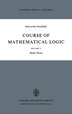Aanmelden
 Aangemeld blijven (i)

De tip van euro-boek.nl
Zoekfuncties
actueel
- 0 resultaten
laagste prijs: € 34,83, hoogste prijs: € 108,43, gemiddelde prijs: € 92,841
bestellen
bij Biblio.co.uk
\$ 41,00
(ongeveer € 34,83)
verzending: € 17,27
bestellen

# Course of Mathematical Logic: Volume 2 Model Theory - gebonden uitgave, pocketboek

1974, ISBN: 9789027702692

Dordrecht, Holland: Springer/Reidel, 1974. Ex-Library. Hardcover. Very Good-/None. Synthese library Dark navy blue cloth boards, blindstamped on front with border and publisher's l… Meer...

Verzendingskosten: EUR 17.27 Quadrant Book Mart
2bestellen
bij ZVAB.com
CHF 114,88
(ongeveer € 106,97)
verzending: € 20,39
bestellen
Course of Mathematical Logic : Volume 2 Model Theory - gebonden uitgave, pocketboek

1974, ISBN: 9027702691

[EAN: 9789027702692], Neubuch, [SC: 20.39], [PU: Springer Netherlands], LOGIK; PHILOSOPHIE / EQUIVALENCE; LEMMA; COMPACTNESSTHEOREM; FORCING; MATHEMATICALLOGIC; MODELTHEORY; PROOF; ULTRAP… Meer...

NEW BOOK. Verzendingskosten: EUR 20.39 AHA-BUCH GmbH, Einbeck, Germany  [Rating: 5 (von 5)]
3bestellen
bij Indigo.ca
C\$ 155,95
(ongeveer € 108,43)
bestellen
Course of Mathematical Logic: Volume 2 Model Theory - nieuw boek

## ISBN: 9789027702692

This book is addressed primarily to researchers specializing in mathemat ical logic. It may also be of interest to students completing a Masters Degree in mathematics and desiring to emba… Meer...

new in stock. Verzendingskosten:zzgl. Versandkosten., exclusief verzendingskosten
4bestellen
bij Achtung-Buecher.de
€ 106,99
verzending: € 0,00
bestellen

Course of Mathematical Logic Volume 2 Model Theory - gebonden uitgave, pocketboek

1974, ISBN: 9027702691

1974 Gebundene Ausgabe Logik, Philosophie / Logik, Mathematische Grundlagen, Philosophie: Logik, Equivalence; Lemma; compactnesstheorem; Forcing; mathematicallogic; Modeltheory; proof; … Meer...

Verzendingskosten:Versandkostenfrei innerhalb der BRD. (EUR 0.00) MARZIES.de Buch- und Medienhandel, 14621 Schönwalde-Glien
5bestellen
bij Hugendubel.de
€ 106,99
verzending: € 0,00
bestellen

Course of Mathematical Logic - gebonden uitgave, pocketboek

ISBN: 9027702691

Course of Mathematical Logic ab 106.99 € als gebundene Ausgabe: Volume 2 Model Theory. Auflage 1974. Aus dem Bereich: Bücher, Wissenschaft, Philosophie, Medien > Bücher, Springer Netherlands

Nr. 4048237. Verzendingskosten:, , DE. (EUR 0.00)

Bijzonderheden over het boek
auteur:

Titel:

ISBN:

## 9789027702692

Gedetalleerde informatie over het boek. - Course of Mathematical Logic: Volume 2 Model Theory

EAN (ISBN-13): 9789027702692
ISBN (ISBN-10): 9027702691
Gebonden uitgave
Verschijningsjaar: 2007
Gewicht: 0,502 kg
Taal: eng/Englisch

Boek bevindt zich in het datenbestand sinds 2007-11-09T23:26:47+01:00 (Amsterdam)
Detailpagina laatst gewijzigd op 2021-10-31T09:21:50+01:00 (Amsterdam)
ISBN/EAN: 9789027702692

ISBN - alternatieve schrijfwijzen:
90-277-0269-1, 978-90-277-0269-2

### Gegevens van de uitgever

Auteur: R. Fraïssé
Titel: Synthese Library; Course of Mathematical Logic - Studies in Epistemology, Logic, Methodology, and Philosophy of Science; Volume 2 Model Theory
Uitgeverij: Springer; Springer Netherland
Verschijningsjaar: 1974-10-31
Dordrecht; NL
Vertaler: David Louvish
Gewicht: 1,090 kg
Taal: Engels
106,99 € (DE)
109,99 € (AT)
118,00 CHF (CH)
POD

BB; Book; Hardcover, Softcover / Philosophie/Allgemeines, Lexika; Philosophie: Logik; Verstehen; Equivalence; Lemma; compactness theorem; forcing; logic; mathematical logic; model theory; proof; ultrapower; ultraproduct; B; Logic; Mathematical Logic and Foundations; Logic; Mathematical Logic and Foundations; Religion and Philosophy; Mathematische Grundlagen; Mathematik: Logik; BC; EA

1/Local Isomorphism and Logical Formula; Logical Restriction Theorem.- 1.1. (k,p)-Isomorphism.- 1.2. (k,p)-Equivalence.- 1.3. Characteristic of a Logical Formula. Relations Between (k,p) -Isomorphism and Logical Formula.- 1.4. Logical Extension and Logical Restriction; Logical Restriction Theorem.- 1.5. Examples of Finitely-Axiomatizable and Non-Finitely-Axiomatizable Multirelations.- 1.6. (k,p)-Interpretability.- 1.7. Homogeneous and Logically Homogeneous Multirelations.- 1.8. Rigid and Logically Rigid Multirelations.- Exercises.- 2/Logical Convergence; Compactness, Omission and Interpretability Theorems.- 2.1. Logical Convergence.- 2.2. Compactness Theorem.- 2.3. Omission Theorem.- 2.4. Interpretability Theorem.- 2.5. Every Injective Logical Operator is Invertible.- Exercise.- 3/Elimination of Quantifiers.- 3.1. Absolute Eliminant.- 3.2. (k,p)-Eliminant.- 3.3. Elimination Algorithms for the Chain of Rational Numbers and the Chain of Natural Numbers.- 3.4. Positive Dense Sum; Elimination of Quantifiers over the Sum of Rational or Real Numbers.- 3.5. Positive Discrete Divisible Sum; Elimination of Quantifiers over the Sum of Natural Numbers.- 3.6. Real Field; Elimination of Quantifiers over the Sum and Product of Algebraic Numbers or Real Numbers.- Exercises.- 4/Extension Theorems.- 4.1. Restrictive Sequence; (k,p)-Isomorphism and (k,p)-Identimorphism.- 4.2. Application to Logical Restriction.- 4.3. Projection Filter.- 4.4. Logical Extension Theorems.- 4.5. Theorem on Common Logical Extensions.- 4.6. Logical Morphism and Logical Embedding.- Exercises.- 5/Theories and Axiom Systems.- 5.1. Theory: Consistency; Intersection of Theories.- 5.1 Axiom System. Class of Models; Union-Theory, Finitely-Axiomatizable Theory, Saturated Theory.- 5.3. Complement of a Theory.- 5.4. Categoricity.- 5.5. Model-Saturated Theory.- Exercises.- 6/Pseudo-Logical Class; Interpretability of Theories; Expansion of a Theory; Axiomatizability.- 6.1. Pseudo-Logical Class.- 6.2. Interpretability of Theories.- 6.3. Canonical Expansion, Semantic Expansion, and Other Expansions.- 6.4. Axiomatizable Multirelations and Theories.- 6.5. Free Expansion.- Exercises.- 7/Ultraproduct.- 7.1. Family of Multirelations, Ultrafilter, Induced Logical Equivalence Class; Ultraproduct and Ultrapower; Maximal Case.- 7.2. Logical Equivalence Implies the Existence of Isomorphic Ultrapowers.- 7.3. Characterization of Logical Classes.- 7.4. Normal Ultraproduct; Definitions and Examples.- 7.5. Normal Ultraproducts and Logical Equivalence.- Exercises.- 8/Forcing.- 8.1. Generic Predicate; System: (+)-Forced and (?)-Forced Formulas.- 8.2. Elementary Properties.- 8.3. Forcing with Constraints.- 8.4. General Relation.- 8.5. Forcing and Deduction; Theory Forced by a Generic Predicate.- Exercises.- 9/Isomorphisms and Equivalences in Relation to the Calculus of Infinitely Long Formulas with Finite Quantifiers.- 9.1. ?-Isomorphism and ?-Equivalence.- 9.2. ?-Isomorphism and ?-Equivalence; Karpian Families.- 9.3. Automorphic Rank of a Multirelation.- 9.4. Multirelations with Denumerable Bases and ?-Isomorphisms.- 9.5. ?-Extension and ?-Interpretability.- 9.6. Infinite Logical Calculi and their Relation to Local Isomorphisms and Equivalences.- Proof of Lemmas Needed to Prove J. Robinson’s Theorem.- Closure of a Relation.- References.

### Laatste soortgelijke boek:9789027705105 Course of Mathematical Logic: Volume 2: Model Theory: Synthese Library No. 69: 002 (R. Frac/Sse, R. Fraossi, R. Fraassa(c))

< naar Archief...